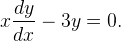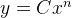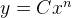# Specific solution of diff eq, no initial value

cowmoo32

## Homework Statement

Consider the differential equationFind a general solution to this differential equation that has the form.
.
.
y = Cx3

Find a second solutionthat might not be a general solution and which may have a different value of n than your first solution.

## The Attempt at a Solution

I solved the first part of the problem
y = Cx3

But am at a complete loss for how to find a second explicit solution without being given an initial value.

Last edited:

## Answers and Replies

Homework Helper
Gold Member
I'm guessing ##y=0## isn't what is being wanted here although it is a solution.

Question: Is ##y = |x|^3## a solution?

Homework Helper
Why would you guess that? $$y= 0x^n$$ is a solution for any n.

Homework Helper
Gold Member
I'm guessing ##y=0## isn't what is being wanted here although it is a solution.

Question: Is ##y = |x|^3## a solution?

Why would you guess that? $$y= 0x^n$$ is a solution for any n.

Are you addressing me? Yes, I know it is a solution for any n, as I said. But, given the poor wording of the question, I thought they might be looking for the more interesting ##y = |x|^3##.

cowmoo32
I've tried y=0 and y=e^c(x^3), I'll see if it accepts y=0x^n but I think they're looking for a specific n

edit: 0x^n was it! Thanks!